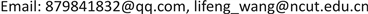1. 引言

2. 四旋翼建模2.1. 运动学方程

R = [ cos ( ψ ) cos ( θ ) sin ( ψ ) cos ( θ ) − sin ( θ )     − sin ( ψ ) cos ( ϕ ) + cos ( ψ ) sin ( θ ) sin ( ϕ ) cos ( ψ ) cos ( ϕ ) + sin ( ψ ) sin ( θ ) sin ( ϕ ) cos ( θ ) sin ( ϕ )     sin ( ψ ) sin ( ϕ ) + cos ( ψ ) sin ( θ ) cos ( ϕ ) − cos ( ψ ) sin ( ϕ ) + sin ( ψ ) sin ( θ ) cos ( ϕ ) cos ( θ ) cos ( ϕ ) ] (1)

P ˙ = R V (2)

Ω ˙ = R w ω (3)

P = ( X , Y , Z ) T 代表四旋翼的位置信息， V = ( U , V , W ) T 是四旋翼在机体坐标系B下的线速度， Ω = ( ϕ , θ , ψ ) T 表示四旋翼的姿态角， ω = ( P , Q , R ) T 为四旋翼机体坐标系B下的角速度。则旋转运动学方程用公式(4)表示。

2.2. 动力学方程

I是四旋翼无人机关于质心的惯性张量矩阵，机体坐标系的原点Ob位于四旋翼的质心，由于四旋翼无人机为一刚体且关于X、Y轴对称，所以 I x y = I x z = I y x = I y z = I z x = I z y = 0 ，惯性矩阵可以转换为式(6)形式。

(6)

3. 动态逆控制器设计3.1. 非线性动态逆

x ˙ = f ( x ) + g ( x ) u y = h ( x ) (7)

y ˙ = ∂ h ∂ x x ˙ = ∂ h ∂ x f ( x ) + ∂ h ∂ x g ( x ) u = F ( x ) + G ( x ) u (8)

3.2. 增量式非线性动态逆

x ̇ = f ( x , u ) (10)

x ˙ ≈ f ( x 0 , u 0 ) + ∂ f ( x , u ) ∂ x | x = x 0 , u = u 0 ( x − x 0 ) + ∂ f ( x , u ) ∂ u | x = x 0 , u = u 0 ( u − u 0 ) x ˙ ≈ x 0 ̇ + F ( x 0 , u 0 ) ( x − x 0 ) + G ( x 0 , u 0 ) ( u − u 0 ) (11)

x ˙ = x ˙ 0 + G ( x 0 , u 0 ) ( u − u 0 ) (12)

u = u 0 + G − 1 ( x 0 , u 0 ) ( υ − x ˙ 0 ) (13)

u k + 1 = u k + G − 1 ( x k , u k ) ( υ k + 1 − x k − x k − 1 Δ t ) (14)

υ k + 1 = x k + 1 − x k Δ t (15)

4. 四旋翼姿态控制器设计4.1. 角度外环

u = G − 1 υ (16)

υ = K a n g e = [ K ϕ ( ϕ d − ϕ ) K θ ( θ d − θ ) K ψ ( ψ d − ψ ) ] (17)

G − 1 = [ 1 0 0   0 cos ( ϕ ) − sin ( ϕ )   − sin s ( θ ) cos ( θ ) sin sin ( ϕ ) cos ( θ ) cos cos ( ϕ ) ] (18)

4.2. 角速度内环

∑ ​ M = M a + M c (19)

M a ∈ R 3 代表气动力距， M c ∈ R 3 表示系统的控制力距。在一个无穷小的时间间隔内，如果控制力据发生很小的变化 Δ M c ，那么角加速度 ω ˙ ∈ R 3 将随之变换，但是气动力距 M a 和角速度 并不会发生变化。这样，可以得到增量形式的表达式

Δ M c = I Δ ω ˙ = I ( ω ˙ d − ω ˙ ) (20)

Δ M c = M c ( Δ u ) (21)

Δ u = M c − 1 I ( υ − ω ˙ ) (22)

υ = K v e l e = [ K p ( P d − P ) K q ( Q d − Q ) K r ( R d − R ) ] (23)

P d , Q d , R d ∈ R 是期望的角速度， P , Q , R ∈ R 是测量的角速度， K v e l ∈ R 3 是比例环节的增益。这样新的控制律中将不再有参数 M a ，不需要建立完整的气动力距方程，降低了对参考模型的依赖程度。图2是完整的姿态控制结构图。

5. 仿真结果

PX4固件支持软件在环仿真和硬件在环仿真两种仿真模式。软件在环仿真可以将完整的飞行控制栈运行在电脑上，通过仿真软件和地面站查看模拟飞行情况和飞行曲线，可以很好的验证改写代码的运行效果。硬件在环仿真是飞控板与仿真软件相连并将模拟固件运行在飞控板上的仿真，它可以测试代码在实际处理器中的运行结果。本文应用增量式非线性动态逆的控制策略对PX4固件进行改写，以Gazebo仿真软件为平台，搭建软件在环仿真的仿真环境，验证控制器在模拟飞行中的飞行效果，仿真数据流如图3所示。

m四旋翼总重1.5 kg
Ixx机体关于x轴的转动惯量0.03 kg/m2
Iyy机体关于y轴的转动惯量0.05 kg/m2
Izz机体关于z轴的转动惯量0.10 kg/m2
Ct旋翼升力系数8.06 * 10−4
Cq旋翼扭矩系数1.0 * 10−6

6. 总结